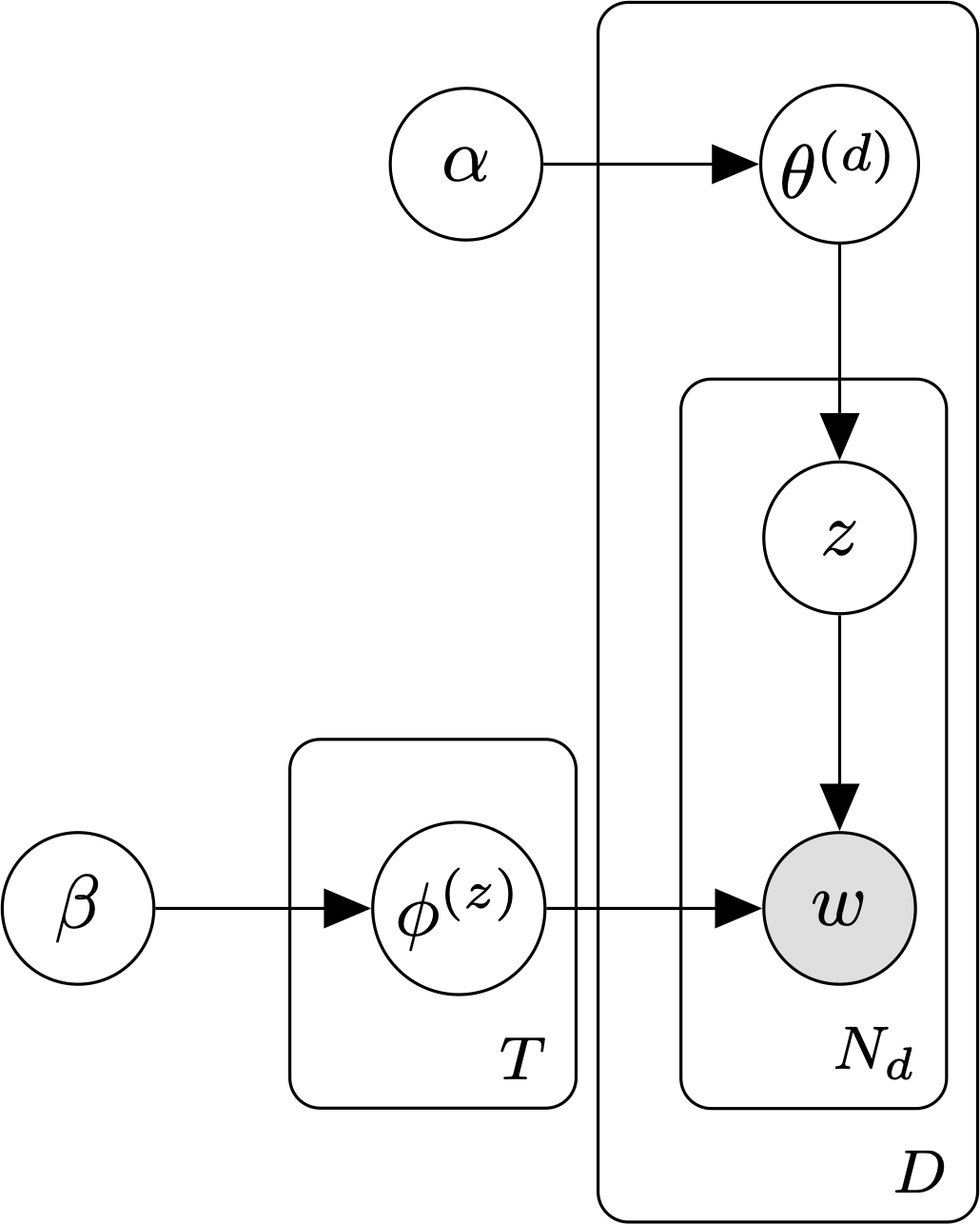# Latent Dirichlet allocation¶

Latent Dirichlet allocation (LDA, commonly known as a topic model) is a generative model for bags of words.The smoothed LDA model with T topics, D documents, and $$N_d$$ words per document.

In LDA, each word in a piece of text is associated with one of T latent topics. A document is an unordered collection (bag) of words. During inference, the goal is to estimate probability of each word token under each topic, along with the per-document topic mixture weights, using only the observed text.

The parameters of the LDA model are:

• $$\theta$$, the document-topic distribution. We use $$\theta^{(i)}$$ to denote the parameters of the categorical distribution over topics associated with document $$i$$.
• $$\phi$$, the topic-word distribution. We use $$\phi^{(j)}$$ to denote the parameters of the categorical distribution over words associated with topic $$j$$.

The standard LDA model  places a Dirichlet prior on $$\theta$$:

$\theta^{(d)} \sim \text{Dir}(\alpha)$

The smoothed/fully-Bayesian LDA model  adds an additional Dirichlet prior on $$\phi$$:

$\phi^{(j)} \sim \text{Dir}(\beta)$

To generate a document with the smoothed LDA model, we:

1. Sample the parameters for the distribution over topics, $$\theta \sim \text{Dir}(\alpha)$$.
2. Sample a topic, $$z \sim \text{Cat}(\theta)$$.
3. If we haven’t already, sample the parameters for topic z’s categorical distribution over words, $$\phi^{(z)} \sim \text{Dir}(\beta)$$.
4. Sample a word, $$w \sim \text{Cat}(\phi^{(z)})$$.
5. Repeat steps 2 through 4 until we have a bag of N words.

The joint distribution over words, topics, $$\theta$$, and $$\phi$$ under the smoothed LDA model is:

$P(w, z, \phi, \theta \mid \alpha, \beta) = \left( \prod_{t=1}^T \text{Dir}(\phi^{(t)}; \beta) \right) \prod_{d=1}^D \text{Dir}(\theta^{(d)}; \alpha) \prod_{n=1}^{N_d} P(z_n \mid \theta^{(d)}) P(w_n \mid \phi^{(z_n)})$

The parameters of the LDA model can be learned using variational expectation maximization or Markov chain Monte Carlo (e.g., collapsed Gibbs sampling).

Models

References

  Blei, D., Ng, A., & Jordan, M. (2003). “Latent Dirichlet allocation”. Journal of Machine Learning Research, 3, 993–1022.
  Griffiths, T. & Steyvers, M. (2004). “Finding scientific topics”. PNAS, 101(1), 5228-5235.